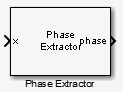# Phase Extractor

Extract the unwrapped phase of a complex input

## Library

Signal Operations

`dspsigops`

•## Description

The Phase Extractor block extracts the unwrapped phase of a complex input. The input can be a vector or matrix. For 2D inputs, the block treats each column as an independent channel. The first dimension is the length of the channel. The second dimension is the number of channels. The block treats 1D inputs as one channel.

The block preserves the input size and dimension, and the output port rate equals the input port rate.

## Examples

This example shows how to use the Phase Extractor block to extract the phase of a sign wave. The DSP Sine Wave block represents the system input signal. Set the DSP Sine Wave block parameters to the following:

• Frequency set to `10` Hz

• set to `Discrete`

• set to `Complex`

• set to `1/1000`

• set to `128`

Do not select the Phase Extractor block parameter .The Time Scope block displays the extracted phase.## Parameters

Unwrap phase only within the frame

When you clear this check box, the block ignores boundaries between the input frames. When you select this check box, the block treats each frame of input data independently, and resets the initial phase value for each new input frame.

Simulate using

Select the simulation type from the following:

• `Code generation`

• `Interpreted execution`

## Supported Data Types

PortSupported Data Types

Input

• Double-precision floating point

• Single-precision floating point

Output

• Double-precision floating point

• Single-precision floating point

 ```dsp.PhaseExtractor ``` DSP System Toolbox

## Algorithms

Consider an input frame of length N:

`$\left(\begin{array}{l}{x}_{1}\\ {x}_{2}\\ ⋮\\ {x}_{N}\end{array}\right)$`

The `step` method acts on this frame and produces this output:

`$\left(\begin{array}{l}{\Phi }_{1}\\ {\Phi }_{2}\\ ⋮\\ {\Phi }_{N}\end{array}\right)$`

where:

`${\Phi }_{i}={\Phi }_{i-1}+\text{angle}\left({x}_{i-1}^{*}{x}_{i}\right)$`

Here, i runs from 1 to N. The `angle` function returns the phase angle in radians.

If the input signal consists of multiple frames:

• If you set `TreatFramesIndependently` to `true`, the `step` method treats each frame independently. Therefore, in each frame, the `step` method calculates the phase using the preceding formula where:

• ${\Phi }_{0}$ is 0.

• ${x}_{0}$ is 1.

• If you set `TreatFramesIndependently` to `false`, the `step` method ignores boundaries between frames. Therefore, in each frame, the `step` method calculates the phase using the preceding formula where:

• ${\Phi }_{0}$ is the last unwrapped phase from the previous frame.

• ${x}_{0}$ is the last sample from the previous frame.

## Extended Capabilities

### C/C++ Code GenerationGenerate C and C++ code using Simulink® Coder™.

Introduced in R2014b

Watch now